# Lions Weekly: March 29, 2023# A Note From Mr. Sandefur

Lion Family,

"When the righteous cry for help, the Lord hears and delivers them out of all their troubles. The Lord is near to the brokenhearted and saves the crushed in spirit." Psalm 34:17-18

I began writing this letter multiple times and though I tried, I could not write to you this week without addressing the events that occurred on Monday in more detail. This week has been a difficult reminder of the world that we live in as we cover our brothers and sisters at Covenant School (Nashville) in prayer. As educators, our deepest priority is to provide a safe and caring environment for each and every student and staff member who calls our campus home. More personally, as a father, I am well aware of the trust that you place in our team daily and that does not go unnoticed or underappreciated.

I have had the honor of speaking with many of you this week as we partner together to ensure the safety of all on our campus. While we have made wonderful strides over the past two years, we continue to seek to add layers of protection, training, and resources in order to establish the environment that we so cherish and seek to protect. I want to assure you that much goes on behind the scenes and we seek to always improve all that we do at The King's Academy, including the area of student and staff safety. I am grateful for your partnership and ask that you please join our faculty and staff in praying for those impacted by the darkest of circumstances and pray that the Lord and His mercy is magnified in this situation and in our nation.

Jeremy Sandefur

 table div table+table+table+table div table{width:100%;padding:0}table div table+table+table+table div table img{width:96.23%;padding:0;float:none}table div table+table+table+table div table td{width:100%;padding:0 1.88% 18px}/* styles */table div table+table+table+table+table+table div table{width:100%;padding:0}table div table+table+table+table+table+table div table img{width:96.23%;padding:0;float:none}table div table+table+table+table+table+table div table td{width:100%;padding:0 1.88% 18px}/* styles */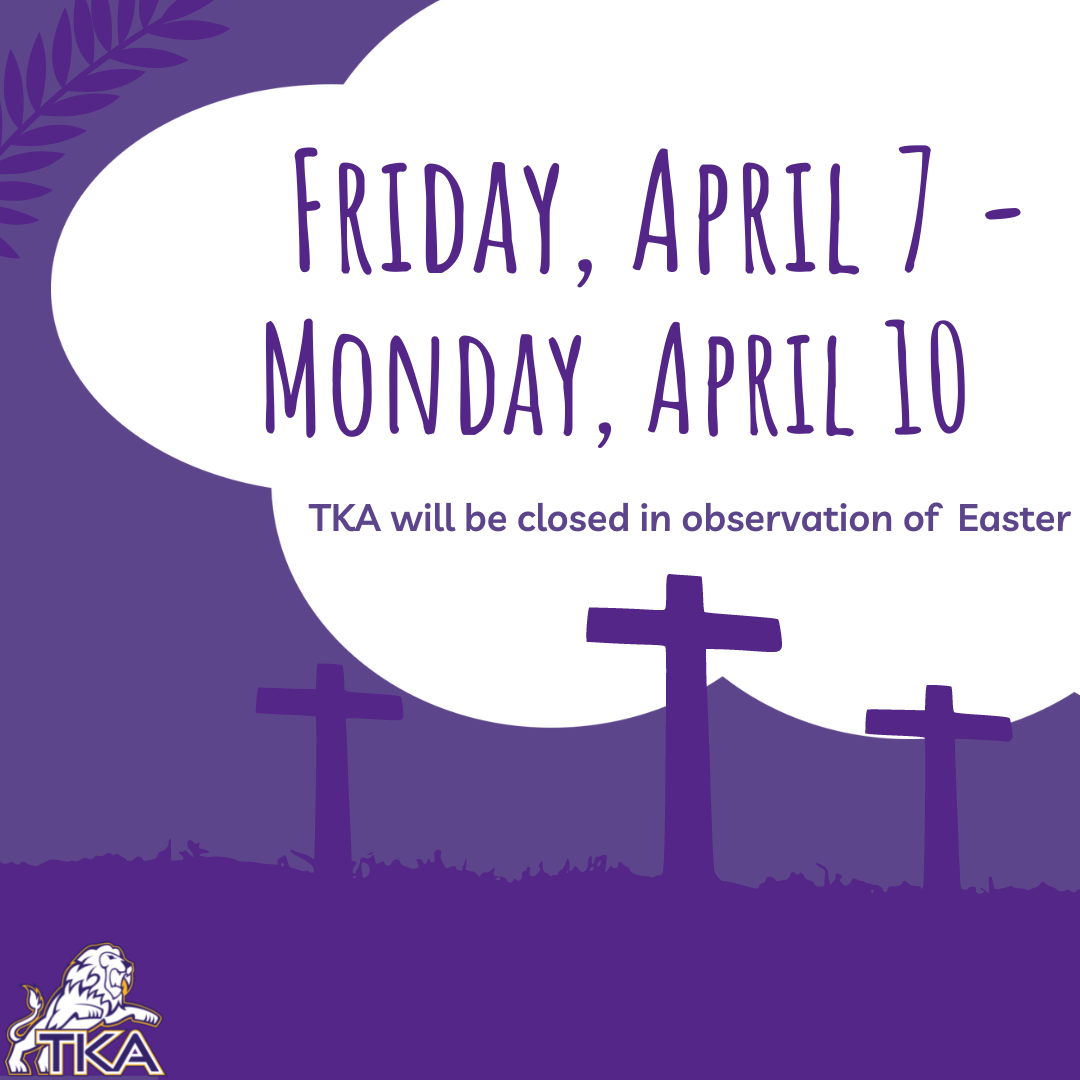# School Closed

TKA school and offices will be closed in observation of Easter on Friday, April 7 and Monday, April 10.

 table div table+table+table+table+table+table+table+table div table{width:100%;padding:0}table div table+table+table+table+table+table+table+table div table img{width:96.23%;padding:0;float:none}table div table+table+table+table+table+table+table+table div table td{width:100%;padding:0 1.88% 18px}/* styles */# Art Showcase

You are cordially invited…
to the MS/HS TKA Art Show!

Students in middle and high school art classes have done an amazing job this year, and they would like to share their work with the community.

TKA artwork will be on display April 3rd-15th at the Vienna Coffee House in downtown Maryville.

Reception and art sales will take place on Saturday, April 15, from 2:00-4:00 pm. Cookies will be provided by PTF at this event.

You may visit the art show anytime during regular coffee house hours. Come and enjoy original artwork by our talented students!

Where: Vienna Coffee House Loft
212 College St. Maryville, 37804

When: April 3rd-15th

Hours: Monday - Saturday, 7 am - 6 pm
Closed on Sundays

 table div table+table+table+table+table+table+table+table+table+table div table{width:100%;padding:0}table div table+table+table+table+table+table+table+table+table+table div table img{width:96.23%;padding:0;float:none}table div table+table+table+table+table+table+table+table+table+table div table td{width:100%;padding:0 1.88% 18px}/* styles */# Used Uniform Sale

## Tuesday, April 11

When: Tuesday, April 11

Time: 3:30 p.m. to 6:00 p.m.

Location: Reagan Pavilion

Contact Person: Sarah Knight 865-621-0741

Who: Anyone is invited to sell or purchase TKA uniforms.

What to Bring: outgrown, new or used polos, pants/shorts/skirts, spirit wear, shoes, backpacks/bags & ANYTHING TKA related. Don’t forget about: Daddy-Daughter & Prom dresses/attire and sport equipment.

What to Expect: We will set up a “uniform shop” under the Reagan Pavilion so you can sell your own items for whatever price you choose. Hopefully we can reuse and repurpose and bless each other. Stay as little or as long as you wish!

SENIORS! Clean out your closets and make a few dollars for college clothes!

If it is not allowed at school, please do not bring it.

 table div table+table+table+table+table+table+table+table+table+table+table+table div table{width:100%;padding:0}table div table+table+table+table+table+table+table+table+table+table+table+table div table img{width:96.23%;padding:0;float:none}table div table+table+table+table+table+table+table+table+table+table+table+table div table td{width:100%;padding:0 1.88% 18px}/* styles */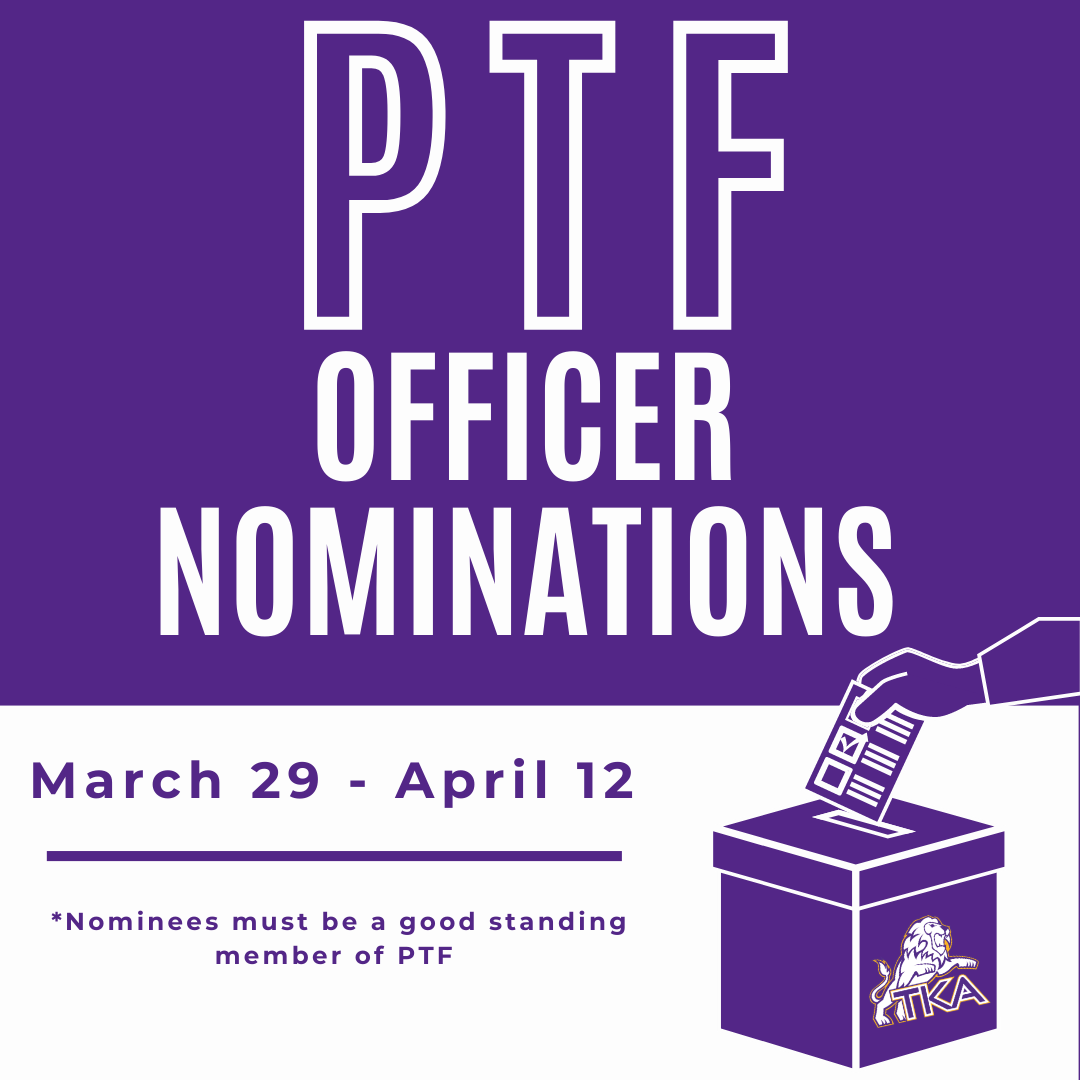# PTF Officer Nominations

## March 29 - April 12

PTF Officer Nominations for the 2023-2024 school year are taking place now through April 12.

Use the link below to nominate a PTF officer.

All officer nominees must be a good standing member of PTF.

 table div table+table+table+table+table+table+table+table+table+table+table+table+table+table+table div table{width:100%;padding:0}table div table+table+table+table+table+table+table+table+table+table+table+table+table+table+table div table img{width:96.23%;padding:0;float:none}table div table+table+table+table+table+table+table+table+table+table+table+table+table+table+table div table td{width:100%;padding:0 1.88% 18px}/* styles */# Elementary Track Meets

Our Elementary Track Team will have two upcoming meets that will take place at CAK. The two meets are on April 12 and 26. The times will be announced at a later date. We hope to see everyone at CAK cheering on our Little Lions!

Dates: April 12 and 26
Location: CAK
Times: TBD

 table div table+table+table+table+table+table+table+table+table+table+table+table+table+table+table+table+table div table{width:100%;padding:0}table div table+table+table+table+table+table+table+table+table+table+table+table+table+table+table+table+table div table img{width:96.23%;padding:0;float:none}table div table+table+table+table+table+table+table+table+table+table+table+table+table+table+table+table+table div table td{width:100%;padding:0 1.88% 18px}/* styles */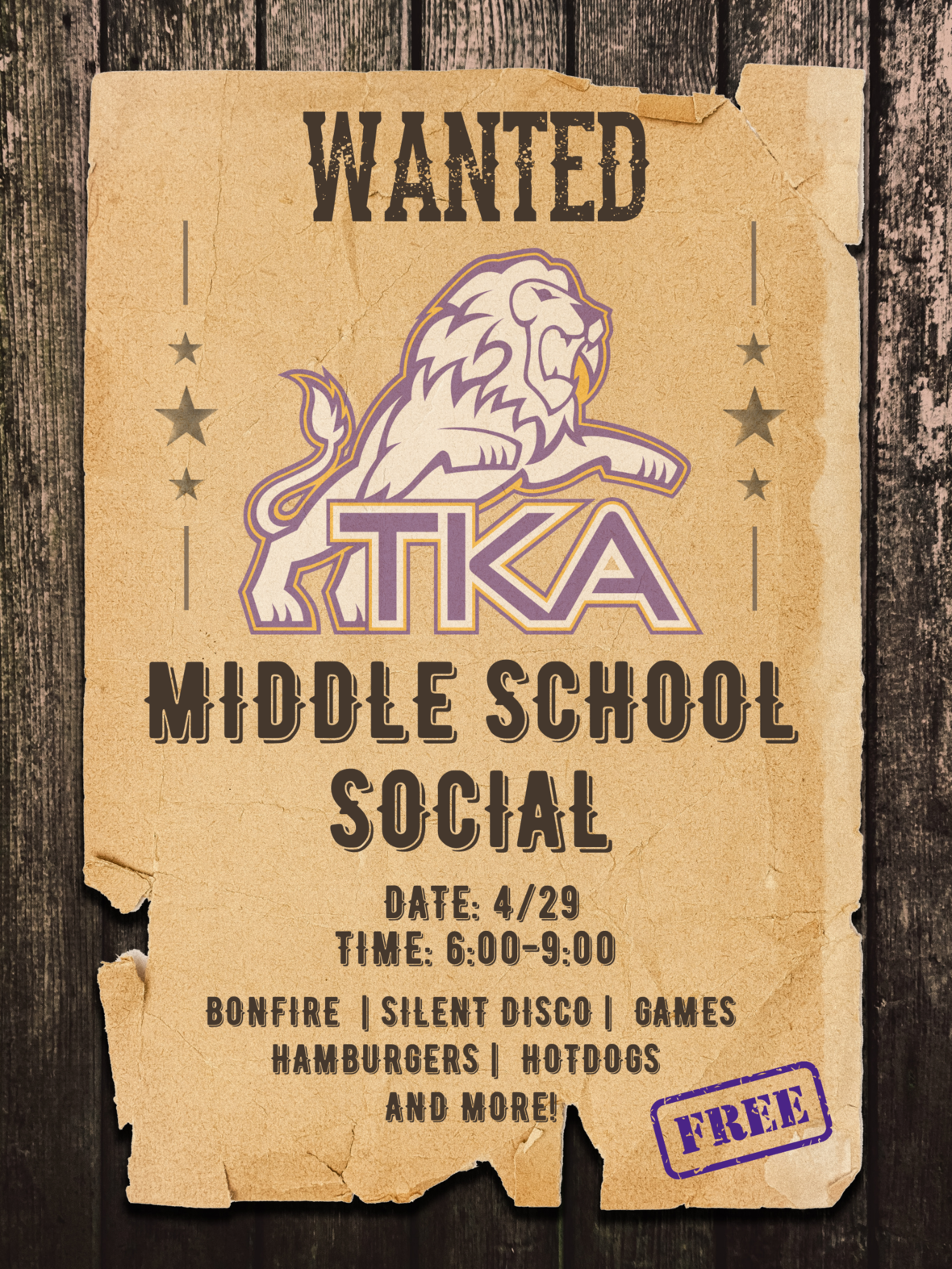# Middle School Social

## Saturday, April 29

Wanted!
Save the Date for TKA's Middle School Social! Saturday, April 29th, from 6:00 p.m.-9:00 p.m. Middle School students are invited to join us for an evening of fun! We will have a bonfire, silent disco, games, food, and more! Keep an eye out for an RSVP form later this week.

 table div table+table+table+table+table+table+table+table+table+table+table+table+table+table+table+table+table+table+table div table{width:100%;padding:0}table div table+table+table+table+table+table+table+table+table+table+table+table+table+table+table+table+table+table+table div table img{width:96.23%;padding:0;float:none}table div table+table+table+table+table+table+table+table+table+table+table+table+table+table+table+table+table+table+table div table td{width:100%;padding:0 1.88% 18px}/* styles */table div table+table+table+table+table+table+table+table+table+table+table+table+table+table+table+table+table+table+table+table+table div table{width:100%;padding:0}table div table+table+table+table+table+table+table+table+table+table+table+table+table+table+table+table+table+table+table+table+table div table img{width:96.23%;padding:0;float:none}table div table+table+table+table+table+table+table+table+table+table+table+table+table+table+table+table+table+table+table+table+table div table td{width:100%;padding:0 1.88% 18px}/* styles */# We're Hiring!

TKA has an employment opportunity available in food services.

Food Services Employee: TKA is seeking an employee to faithfully serve in our food services department. Prior food service experience is preferred. Please email resumes to HR@thekingsacademy.net or call 865-573-8321.

 table div table+table+table+table+table+table+table+table+table+table+table+table+table+table+table+table+table+table+table+table+table+table+table+table div table{width:100%;padding:0}table div table+table+table+table+table+table+table+table+table+table+table+table+table+table+table+table+table+table+table+table+table+table+table+table div table img{width:96.23%;padding:0;float:none}table div table+table+table+table+table+table+table+table+table+table+table+table+table+table+table+table+table+table+table+table+table+table+table+table div table td{width:100%;padding:0 1.88% 18px}/* styles */# Prom Information

DATE: April 1, 2023
PLACE: Capitol Theatre, Maryville, TN
TIME: 6:00 pm - 10:30 pm

# Ticket Information

Tickets for this year's High School Prom are on sale now!

COST: \$55 per person from March 18-March 31

## How to purchase tickets:

▪ The student must enter the promotional code: TKA37865
▪ Maximum 2 tickets per order
▪ After selecting the number of tickets to purchase, you must enter Additional Information on the 2nd page before completing the purchase.
 ▪ The student must enter the promotional code: TKA37865
 ▪ Maximum 2 tickets per order
 ▪ After selecting the number of tickets to purchase, you must enter Additional Information on the 2nd page before completing the purchase.

# Prom Details

DATE: April 1, 2023
PLACE: Capitol Theatre, Maryville, TN
TIME: 6:00 pm - 10:30 pm
COST: \$50 per person through March 17/\$55 per person March 18-March 31.

Desserts/Light Refreshments will be served.

OUTSIDE GUESTS:
ALL outside guests must be approved. An outside guest form can be found in Mrs. Griffin's office or by using the link below.

DRESS CODE REQUIREMENTS:
Girls - Prom and homecoming dresses must be viewed on the individual in advance for suitable fitting - no exceptions. Skirts of the dresses may not be sheer (see through) material. “Dollar Bill” test will be used for the length. Length should be no shorter than width of dollar bill above knee (approximately two inches). Neckline and backline must be modest (backline must be above the natural waistline). Spaghetti straps and strapless styles are acceptable, if modest. The midriff should always remain covered. No open sides or sheer panels. No immodest tight dresses are allowed. Viewing dates will be provided for both homecoming and prom.

Boys - Suit & Tie or Tuxedo, Dress Shoes

PROM DRESS CHECKS:
Email to lwallace@thekingsacademy.net and kpolk@thekingsacademy.net - send pics of front and back full length of dress in good lighting.

Remember, all attire must adhere to dress code.

## Contact Jenny Cook via text 865-387-3468 with any prom related questions.

 table div table+table+table+table+table+table+table+table+table+table+table+table+table+table+table+table+table+table+table+table+table+table+table+table+table+table+table+table+table+table div table{width:100%;padding:0}table div table+table+table+table+table+table+table+table+table+table+table+table+table+table+table+table+table+table+table+table+table+table+table+table+table+table+table+table+table+table div table img{width:96.23%;padding:0;float:none}table div table+table+table+table+table+table+table+table+table+table+table+table+table+table+table+table+table+table+table+table+table+table+table+table+table+table+table+table+table+table div table td{width:100%;padding:0 1.88% 18px}/* styles */table div table+table+table+table+table+table+table+table+table+table+table+table+table+table+table+table+table+table+table+table+table+table+table+table+table+table+table+table+table+table+table+table div table{width:100%;padding:0}table div table+table+table+table+table+table+table+table+table+table+table+table+table+table+table+table+table+table+table+table+table+table+table+table+table+table+table+table+table+table+table+table div table img{width:96.23%;padding:0;float:none}table div table+table+table+table+table+table+table+table+table+table+table+table+table+table+table+table+table+table+table+table+table+table+table+table+table+table+table+table+table+table+table+table div table td{width:100%;padding:0 1.88% 18px}/* styles */Lion Family,

I pray that each of you is doing well and had a blessed week off for Spring Break. It is hard to imagine that the end of our academic year is only eight weeks away, and from experience, I know that we will find ourselves at Graduation in a hurry.

While we are still working hard to finish this semester with excellence, our Leadership Team continues to pray and prepare for the upcoming school year. As we make such preparations, it is my desire to inform you of some changes that are occurring within our Admissions Team.

It is in such a bittersweet spirit that I inform you of the retirement of Ms. Janice Mink. Ms. Mink has done more for the ministry of The King's Academy than anyone I have met during my time on campus. She has led so faithfully and has agreed to continue serving until the end of December. Please join me in thanking Ms. Mink for her hard work, dedication, and the impact that she has had on countless lives.

While we will greatly miss Ms. Mink, though we have made her promise to stay involved and engaged with our students and staff, we are honored to announce how the Lord is moving in our Admissions Team. Please join me in welcoming the new members of our Admissions Team to their roles as we continue to seek the harvest that the Lord has provided internationally for our school community.

In Christ Alone,

Jeremy Sandefur# Janice Mink - Retirement

Ms. Janice Mink will be retiring at the end of December 2023. Ms. Mink has faithfully served HCBA/TKA for forty years as a dedicated and impactful member of our school leadership team, overseeing the admissions process for hundreds of international students. Ms. Mink is an encouragement to everyone she comes in contact with both locally and internationally. The legacy that Ms. Mink leaves behind is one of godly wisdom, kindness, discernment, inclusivity, and a loving heart for the nations.

Ms. Mink has graciously agreed to pour into our new leaders and serve until the end of the calendar year. When you see Ms. Mink, please thank her and let her know how she has impacted your life.# April Kandel - Director of International Admissions

April Kandel has served our TKA family in the capacity of a high school math and Bible teacher since 2014. April has imparted her love of numbers and Jesus and His kingdom realities to many here at The King’s Academy. Her passion is that all may know and experience the life-changing gospel of Jesus Christ.

April has served 6 years as an international missionary with her husband of 28 years, John Kandel. April holds a B.S. in Business marketing and public relations as well as a Master’s of Education. She has served as a teacher since 1998 in various Christian schools and public schools as well as built her own wellness business. She is a Bible teacher/public speaker throughout our community and surrounding areas. April and John have 4 wonderful sons, 2 daughter-in-laws, and one grandson with grandbaby number 2 on the way. Her 4th son, Elijah Kandel, is currently a Junior here at TKA.

As April transitions to become our next Director of International Admissions, she prays that she is able to be a light of truth and hope to those families that will become a part of our TKA family as we seek to offer those families a place with a safe community that fosters an environment for experiencing the love of God, witnessing what it means to be a servant leader, and learning to live from a place of overall excellence. We are grateful for Mrs. Kandel's impact thus far at TKA and are looking forward to her service in this new area.# Greg Gibson - International Outreach Consultant

Greg Gibson joins our Admissions Team as our International Outreach Consultant. Gregory Gibson is a global church planting catalyst, gospel entrepreneur, and the founder of Gibson Leadership. Most importantly, he is married to Grace, and he is the proud father of Cora and Iver who are TKA students.

Gibson's role will be to work as the intermediary between TKA and students throughout the globe. Gibson looks forward to partnering with our Admissions Team to identify students that will be good fits for The Academy and to ensure that our International Boarding program is able to continue to grow and meet the needs of our school community.

 table div table+table+table+table+table+table+table+table+table+table+table+table+table+table+table+table+table+table+table+table+table+table+table+table+table+table+table+table+table+table+table+table+table+table+table+table+table div table{width:100%;padding:0}table div table+table+table+table+table+table+table+table+table+table+table+table+table+table+table+table+table+table+table+table+table+table+table+table+table+table+table+table+table+table+table+table+table+table+table+table+table div table img{width:96.23%;padding:0;float:none}table div table+table+table+table+table+table+table+table+table+table+table+table+table+table+table+table+table+table+table+table+table+table+table+table+table+table+table+table+table+table+table+table+table+table+table+table+table div table td{width:100%;padding:0 1.88% 18px}/* styles */# D1 Training

Middle/High school athletes can come train outside of their team training hours at the listed times.

For any additional information, parents can contact Mr. Melvin Jones.

Melvin Jones
D1 Sevierville
865-719-9421
melvin.jones@d1training.com

 table div table+table+table+table+table+table+table+table+table+table+table+table+table+table+table+table+table+table+table+table+table+table+table+table+table+table+table+table+table+table+table+table+table+table+table+table+table+table+table div table{width:100%;padding:0}table div table+table+table+table+table+table+table+table+table+table+table+table+table+table+table+table+table+table+table+table+table+table+table+table+table+table+table+table+table+table+table+table+table+table+table+table+table+table+table div table img{width:96.23%;padding:0;float:none}table div table+table+table+table+table+table+table+table+table+table+table+table+table+table+table+table+table+table+table+table+table+table+table+table+table+table+table+table+table+table+table+table+table+table+table+table+table+table+table div table td{width:100%;padding:0 1.88% 18px}/* styles */# 2022-23 Yearbooks

2022-23 yearbooks are now on sale! Scan this QR code to visit the site to purchase yours today. For any questions regarding yearbooks, contact Mr. Josh Smith at jsmith@thekingsacademy.net.

 table div table+table+table+table+table+table+table+table+table+table+table+table+table+table+table+table+table+table+table+table+table+table+table+table+table+table+table+table+table+table+table+table+table+table+table+table+table+table+table+table+table div table{width:100%;padding:0}table div table+table+table+table+table+table+table+table+table+table+table+table+table+table+table+table+table+table+table+table+table+table+table+table+table+table+table+table+table+table+table+table+table+table+table+table+table+table+table+table+table div table img{width:96.23%;padding:0;float:none}table div table+table+table+table+table+table+table+table+table+table+table+table+table+table+table+table+table+table+table+table+table+table+table+table+table+table+table+table+table+table+table+table+table+table+table+table+table+table+table+table+table div table td{width:100%;padding:0 1.88% 18px}/* styles */# Middle School Golf Update

Our middle school golf teams are off to a great start! The Lady Lions won their match Monday against Northview, and Camdyn Cole placed 1st individually! Way to go Lions!

 table div table+table+table+table+table+table+table+table+table+table+table+table+table+table+table+table+table+table+table+table+table+table+table+table+table+table+table+table+table+table+table+table+table+table+table+table+table+table+table+table+table+table+table div table{width:100%;padding:0}table div table+table+table+table+table+table+table+table+table+table+table+table+table+table+table+table+table+table+table+table+table+table+table+table+table+table+table+table+table+table+table+table+table+table+table+table+table+table+table+table+table+table+table div table img{width:96.23%;padding:0;float:none}table div table+table+table+table+table+table+table+table+table+table+table+table+table+table+table+table+table+table+table+table+table+table+table+table+table+table+table+table+table+table+table+table+table+table+table+table+table+table+table+table+table+table+table div table td{width:100%;padding:0 1.88% 18px}/* styles */# Weekly Sports Scheduletable div table+table+table+table+table+table+table+table+table+table+table+table+table+table+table+table+table+table+table+table+table+table+table+table+table+table+table+table+table+table+table+table+table+table+table+table+table+table+table+table+table+table+table+table+table+table div table{width:100%;padding:0}table div table+table+table+table+table+table+table+table+table+table+table+table+table+table+table+table+table+table+table+table+table+table+table+table+table+table+table+table+table+table+table+table+table+table+table+table+table+table+table+table+table+table+table+table+table+table div table img{width:96.23%;padding:0;float:none}table div table+table+table+table+table+table+table+table+table+table+table+table+table+table+table+table+table+table+table+table+table+table+table+table+table+table+table+table+table+table+table+table+table+table+table+table+table+table+table+table+table+table+table+table+table+table div table td{width:100%;padding:0 1.88% 18px}/* styles */# Important information for the 2022-2023 School Year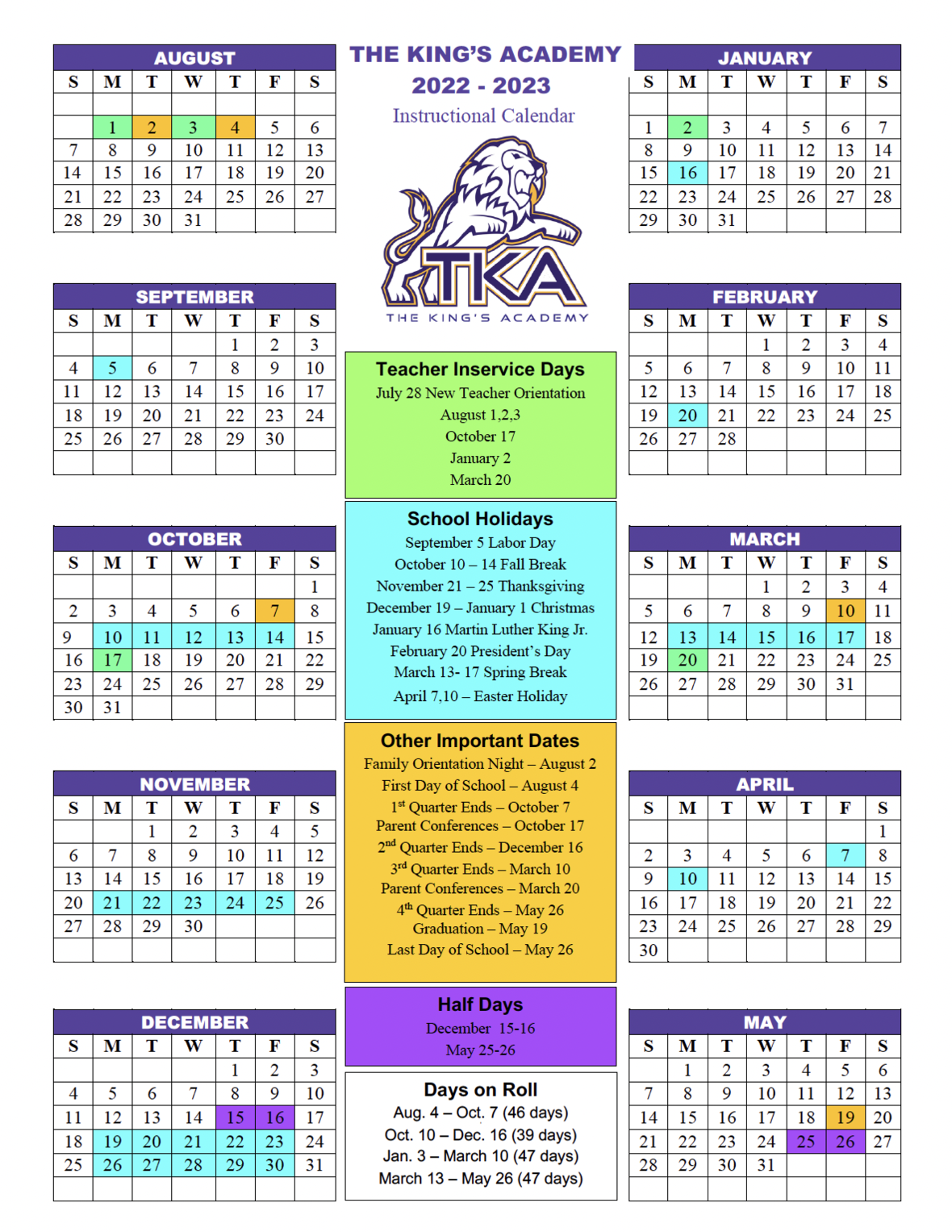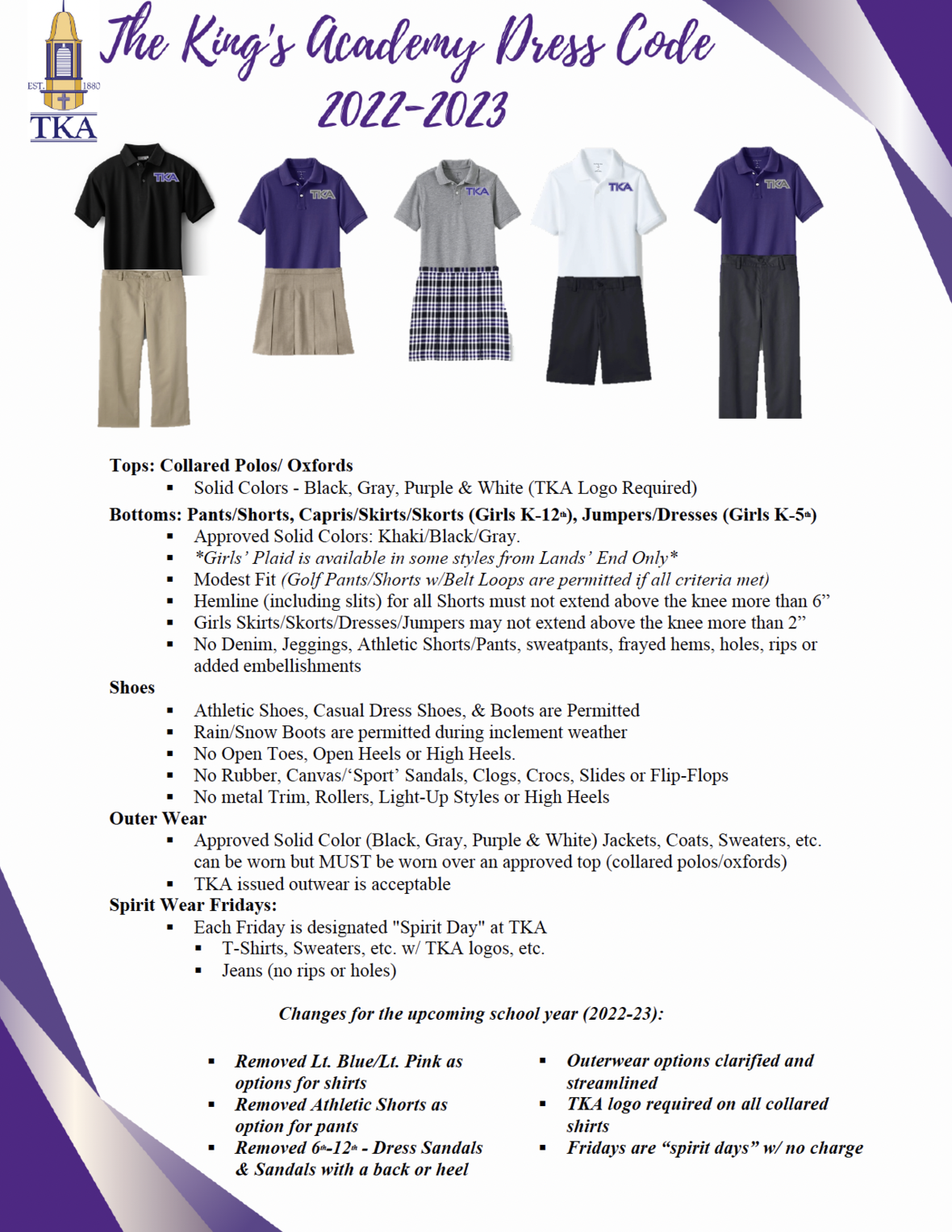table div table+table+table+table+table+table+table+table+table+table+table+table+table+table+table+table+table+table+table+table+table+table+table+table+table+table+table+table+table+table+table+table+table+table+table+table+table+table+table+table+table+table+table+table+table+table+table+table+table+table+table div table{width:100%;padding:0}table div table+table+table+table+table+table+table+table+table+table+table+table+table+table+table+table+table+table+table+table+table+table+table+table+table+table+table+table+table+table+table+table+table+table+table+table+table+table+table+table+table+table+table+table+table+table+table+table+table+table+table div table img{width:96.23%;padding:0;float:none}table div table+table+table+table+table+table+table+table+table+table+table+table+table+table+table+table+table+table+table+table+table+table+table+table+table+table+table+table+table+table+table+table+table+table+table+table+table+table+table+table+table+table+table+table+table+table+table+table+table+table+table div table td{width:100%;padding:0 1.88% 18px}/* styles */# TKA Visitor Policy

In order to help maintain a safe and instructionally based learning environment for our students, all visitors for middle and high school must enter through the front door of the Anderson Building and sign in in the middle/high school office in order to receive a visitor's pass. All visitors for elementary must enter the front door of the Ogle Building in order to receive a visitor's pass. This pass should be visible at all times so that TKA faculty and staff know that you have checked in at the office.

Our doors are open to visitors, but please call ahead of time to schedule conferences with your child’s teachers. This will prevent interruptions to instruction and the loss of the valuable learning time of our students. Adults will not be allowed to wander the halls of the building or hold conferences with teachers during instructional times.

TKA family lunch visitors should follow the above procedure. Lunch tickets are \$5 each.

Students who are not enrolled at TKA are not permitted to visit TKA students during the school day, which includes lunch.

This policy has been developed in order to help maintain a safe and instruction focused environment at school each day. Any visitor who does not meet these expectations may be asked to leave school grounds.

 table div table+table+table+table+table+table+table+table+table+table+table+table+table+table+table+table+table+table+table+table+table+table+table+table+table+table+table+table+table+table+table+table+table+table+table+table+table+table+table+table+table+table+table+table+table+table+table+table+table+table+table+table+table div table{width:100%;padding:0}table div table+table+table+table+table+table+table+table+table+table+table+table+table+table+table+table+table+table+table+table+table+table+table+table+table+table+table+table+table+table+table+table+table+table+table+table+table+table+table+table+table+table+table+table+table+table+table+table+table+table+table+table+table div table img{width:96.23%;padding:0;float:none}table div table+table+table+table+table+table+table+table+table+table+table+table+table+table+table+table+table+table+table+table+table+table+table+table+table+table+table+table+table+table+table+table+table+table+table+table+table+table+table+table+table+table+table+table+table+table+table+table+table+table+table+table+table div table td{width:100%;padding:0 1.88% 18px}/* styles *//* styles */
 table div table+table+table+table+table+table+table+table+table+table+table+table+table+table+table+table+table+table+table+table+table+table+table+table+table+table+table+table+table+table+table+table+table+table+table+table+table+table+table+table+table+table+table+table+table+table+table+table+table+table+table+table+table+table+table+table div table{width:100%;padding:0}table div table+table+table+table+table+table+table+table+table+table+table+table+table+table+table+table+table+table+table+table+table+table+table+table+table+table+table+table+table+table+table+table+table+table+table+table+table+table+table+table+table+table+table+table+table+table+table+table+table+table+table+table+table+table+table+table div table img{width:96.23%;padding:0;float:none}table div table+table+table+table+table+table+table+table+table+table+table+table+table+table+table+table+table+table+table+table+table+table+table+table+table+table+table+table+table+table+table+table+table+table+table+table+table+table+table+table+table+table+table+table+table+table+table+table+table+table+table+table+table+table+table+table div table td{width:100%;padding:0 1.88% 18px}/* styles */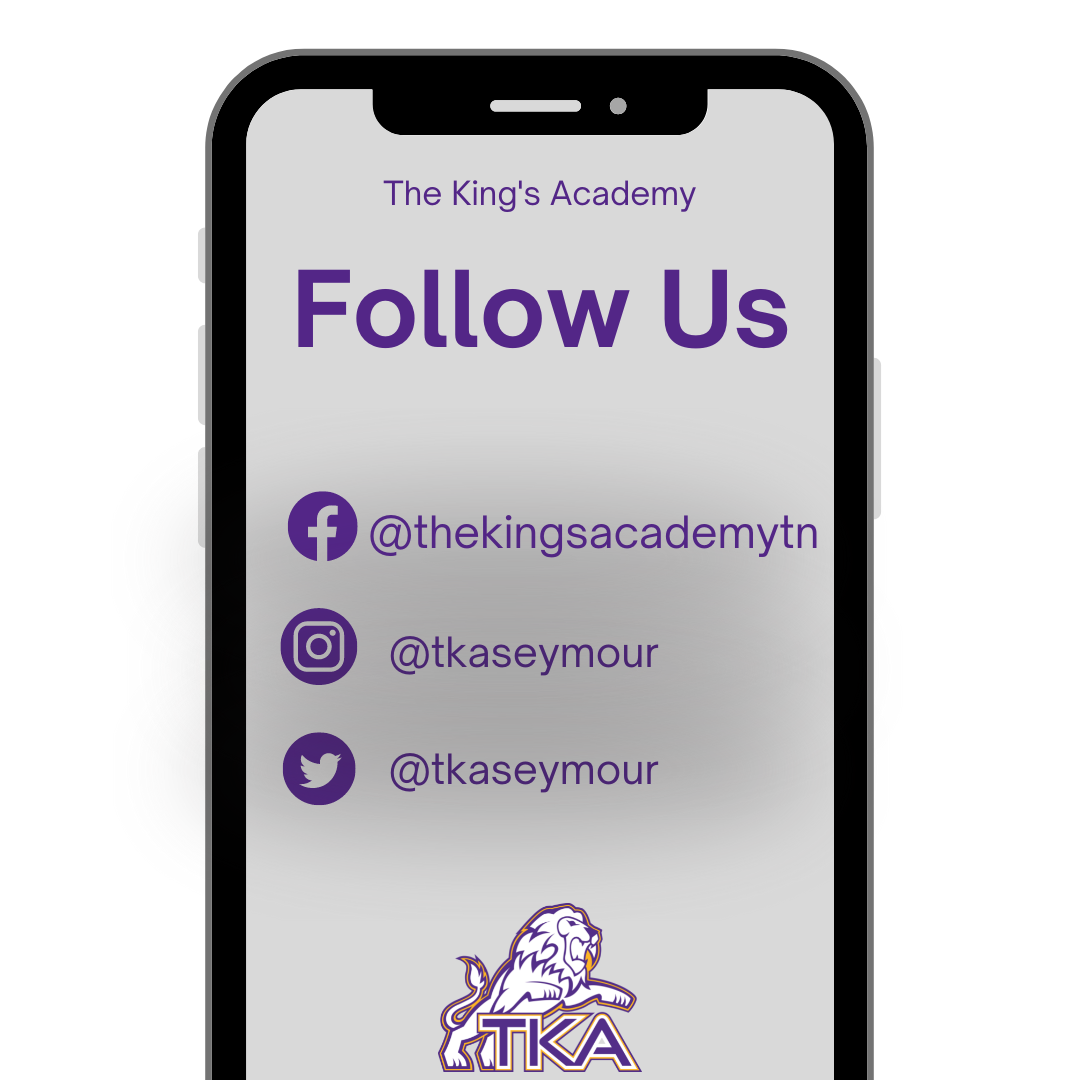table div table+table+table+table+table+table+table+table+table+table+table+table+table+table+table+table+table+table+table+table+table+table+table+table+table+table+table+table+table+table+table+table+table+table+table+table+table+table+table+table+table+table+table+table+table+table+table+table+table+table+table+table+table+table+table+table+table+table div table{width:100%;padding:0}table div table+table+table+table+table+table+table+table+table+table+table+table+table+table+table+table+table+table+table+table+table+table+table+table+table+table+table+table+table+table+table+table+table+table+table+table+table+table+table+table+table+table+table+table+table+table+table+table+table+table+table+table+table+table+table+table+table+table div table img{width:96.23%;padding:0;float:none}table div table+table+table+table+table+table+table+table+table+table+table+table+table+table+table+table+table+table+table+table+table+table+table+table+table+table+table+table+table+table+table+table+table+table+table+table+table+table+table+table+table+table+table+table+table+table+table+table+table+table+table+table+table+table+table+table+table+table div table td{width:100%;padding:0 1.88% 18px}/* styles *//* styles */ The King's Academy
 Like   Tweet